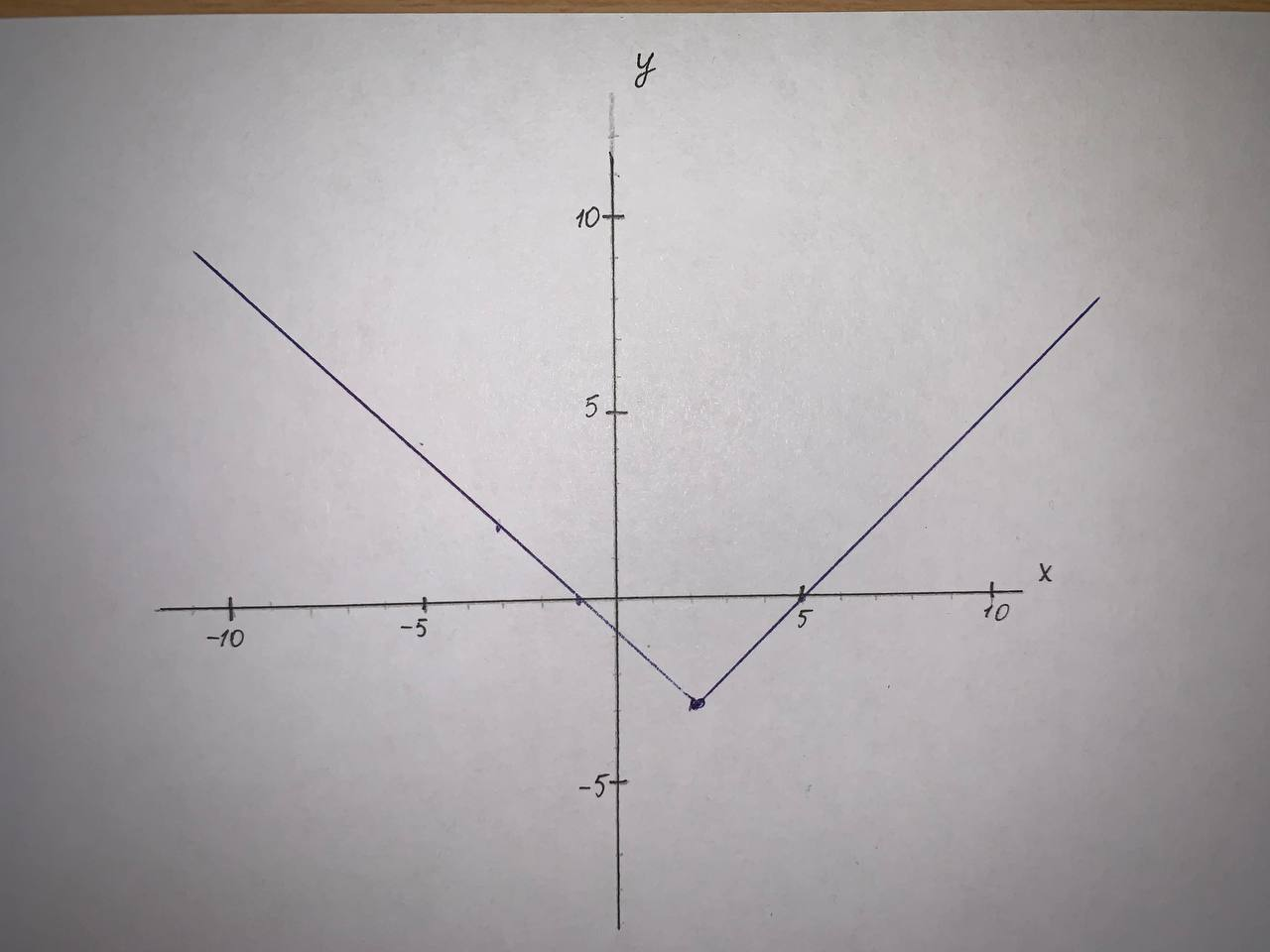# Recent questions in College algebra

Recent questions in College algebraTobias Ali 2021-09-08 Answered

### Graph the function by hand, not by plotting points, but by starting with the graph of one of the standard functions and then applying the appropriate transformations. $$\displaystyle{y}={1}-{2}{x}+{x}^{{2}}$$preprekomW 2021-09-07 Answered

### Write an equation for the graphed function by using transformations of the graphs of one of the toolkit functions. y=?tinfoQ 2021-08-21 Answered

### By using the transformation of function $$\displaystyle{y}={x}^{{2}}$$, sketch the function of y=$$\displaystyle{\frac{{{\left({x}-{2}\right)}^{{2}}}}{{{3}}}}+{4}$$Ramsey 2021-08-20 Answered

### By using the transformation of function $$\displaystyle{y}={\cos{{x}}}$$, sketch the function of $$\displaystyle{y}=-{\cos{{\left({3}{x}-\frac{\pi}{{6}}\right)}}}+{4}$$banganX 2021-08-20 Answered

### By using the transformation of function y=|x|, sketch the function of y=|x-3|+2Armorikam 2021-08-17 Answered

### By using the transformations of function $$\displaystyle{y}={x}^{{2}}$$, sketch the function of $$\displaystyle{y}={x}{\left({6}+{x}\right)}{)}$$Tabansi 2021-08-15 Answered

### By using the transformation of function $$\displaystyle{y}={\sin{{x}}}$$, sketch the function y=$$\displaystyle{3}\cdot{\sin{{\left(\frac{{x}}{{2}}\right)}}}$$Jaden Easton 2021-08-14 Answered

### What transformations of the parent graph of $$\displaystyle{f{{\left({x}\right)}}}=\sqrt{{c}}$$ produce the graphs of the following functions? a) $$\displaystyle{m}{\left({x}\right)}=\sqrt{{{7}{x}-{3.5}}}-{10}$$ b) $$\displaystyle{j}{\left({x}\right)}=-{2}\sqrt{{{12}{x}}}+{4}$$aortiH 2021-08-10 Answered

### Begin by graphing $$\displaystyle{f{{\left({x}\right)}}}={{\log}_{{{2}}}{x}}$$ Then use transformations of this graph to graph the given function. What is the graph's x-intercept? What is the vertical asymptote? Use the graphs to determine each function's domain and range. $$\displaystyle{g{{\left({x}\right)}}}={{\log}_{{{2}}}{\left({x}-{2}\right)}}$$snowlovelydayM 2021-08-10 Answered

### Sketch a graph of the function. Use transformations of functions when ever possible. $$\displaystyle{f{{\left({x}\right)}}}={1}+\sqrt{{{x}}}$$kuCAu 2021-08-09 Answered

### Graph f and g in the same rectangular coordinate system. Use transformations of the graph of f to obtain the graph of g. Graph and give equations of all asymptotes Use the graphs to determine each function's domain and range. $$\displaystyle{f{{\left({x}\right)}}}={2}^{{{x}}}\ {\quad\text{and}\quad}\ {g{{\left({x}\right)}}}={2}^{{{x}-{1}}}$$Annette Arroyo 2021-08-08 Answered

### Begin by graphing $$\displaystyle{f{{\left({x}\right)}}}={{\log}_{{{2}}}{x}}$$. Then use transformations of this graph to graph the given function. What is the graph's x-intercept? What is the vertical asymptote? Use the graphs to determine each function's domain and range. $$\displaystyle{h}{\left({x}\right)}=-{1}+{{\log}_{{{2}}}{x}}$$Burhan Hopper 2021-08-08 Answered

### Describe the transformations and sketch the graphs of the following trigonometric functions. $$\displaystyle{a}.{f{{\left({x}\right)}}}=-{2}{\cos{{\left({2}{x}\right)}}}+{3}\\ {b}.{g{{\left({x}\right)}}}={3}{\sin{{\left({x}-\pi\right)}}}-{1}$$tricotasu 2021-08-05 Answered

### Begin by graphing $$f(x)= \log_{2}x.$$ Then use transformations of this graph to graph the given function. What is the graph's x-intercept? What is the vertical asymptote? Use the graphs to determine each functions domain and range. $$\displaystyle{h}{\left({x}\right)}=-{1}+{{\log}_{{{2}}}{x}}$$tabita57i 2021-08-04 Answered

### Graph f and g in the same rectangular coordinate system. Use transformations of the graph of f to obtain the graph of g. Graph and give equations of all asymptotes. Use the graphs to determine each function's domain and range. $$\displaystyle{f{{\left({x}\right)}}}={\log{{x}}}\ {\quad\text{and}\quad}\ {g{{\left({x}\right)}}}=-{\log{{\left({x}+{3}\right)}}}$$Joni Kenny 2021-08-03 Answered

### Graph f and g in the same rectangular coordinate system. Use transformations of the graph of f to obtain the graph of g. Graph and give equations of all asymptotes. Use the graphs to determine each function's domain arid range. $$\displaystyle{f{{\left({x}\right)}}}={e}^{{{x}}}{\quad\text{and}\quad}{g{{\left({x}\right)}}}={2}{e}^{{{\frac{{{x}}}{{{2}}}}}}$$Tammy Todd 2021-08-01 Answered

### Begin by graphing $$\displaystyle{f{{\left({x}\right)}}}={\log{{2}}}{x}$$ Then use transformations of this graph to graph the given function. What is the graph's x-intercept? What is the vertical asymptote? Use the graphs to determine each functions domain and range. $$\displaystyle{r}{\left({x}\right)}={{\log}_{{{2}}}{\left(-{x}\right)}}$$Brennan Flores 2021-07-30 Answered

### Graph f and g in the same rectangular coordinate system. Use transformations of the graph of f to obtain the graph of g. Graph and give equations of all asymptotes. Use the graphs to determine each function's domain and range. $$\displaystyle{f{{\left({x}\right)}}}={\left({\frac{{{1}}}{{{2}}}}\right)}^{{{x}}}\ {\quad\text{and}\quad}\ {g{{\left({x}\right)}}}={\left({\frac{{{1}}}{{{2}}}}\right)}^{{-{x}}}$$CoormaBak9 2021-07-30 Answered

### For $$\displaystyle{y}={3}+{{\log}_{{{2}}}{x}}$$. a) Use transformations of the graphs of $$\displaystyle{y}={{\log}_{{{2}}}{x}}$$ and $$\displaystyle{y}={{\log}_{{{3}}}{x}}$$ o graph the given functions. b) Write the domain and range in interval notation. c) Write an equation of the asymptote.Tazmin Horton 2021-07-04 Answered

...